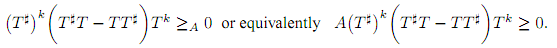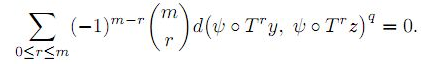The Australian Journal of Mathematical Analysis and Applications

 Home News Editors Volumes RGMIA Subscriptions Authors Contact

ISSN 1449-5910

You searched for mahmoud
Total of 7 results found in site

3: Paper Source PDF document

Paper's Title:

Hyponormal and K-Quasi-Hyponormal Operators On Semi-Hilbertian Spaces

Author(s):

Ould Ahmed Mahmoud Sid Ahmed and Abdelkader Benali

Mathematics Department,
College of Science,
Aljouf University,
Aljouf 2014,
Saudi Arabia.
E-mail: sididahmed@ju.edu.sa

Mathematics Department, Faculty of Science,
Hassiba Benbouali, University of Chlef,
B.P. 151 Hay Essalem, Chlef 02000,
Algeria.
E-mail: benali4848@gmail.com

Abstract:

Let H be a Hilbert space and let A be a positive bounded operator on H. The semi-inner product < u|v>A:=<Au|v>, u,v H induces a semi-norm || .||A on H. This makes H into a semi-Hilbertian space. In this paper we introduce the notions of hyponormalities and k-quasi-hyponormalities for operators on semi Hilbertian space (H,||.||A), based on the works that studied normal, isometry, unitary and partial isometries operators in these spaces. Also, we generalize some results which are already known for hyponormal and quasi-hyponormal operators. An operator T BA (H) is said to be (A, k)-quasi-hyponormal if2: Paper Source PDF document

Paper's Title:

ψ(m,q)-Isometric Mappings on Metric Spaces

Author(s):

Sid Ahmed Ould Beinane, Sidi Hamidou Jah and Sid Ahmed Ould Ahmed Mahmoud

Mathematical Analysis and Applications, Mathematics Department, College of Science,
Jouf University,
Sakaka P.O.Box 2014,
Saudi Arabia.
E-mail: beinane06@gmail.com

Department of Mathematics, College of Science Qassim University,
P.O. Box 6640, Buraydah 51452,
Saudi Arabia.
E-mail: jahsiidi@yahoo.fr

Mathematical Analysis and Applications,
Mathematics Department, College of Science, Jouf University,
Sakaka P.O.Box 2014,
Saudi Arabia.
E-mail: sidahmed@ju.edu.sa, sidahmed.sidha@gmail.com

Abstract:

The concept of (m,p)-isometric operators on Banach space was extended to (m,q)-isometric mappings on general metric spaces in . This paper is devoted to define the concept of ψ(m, q)-isometric, which is the extension of A(m, p)-isometric operators on Banach spaces introduced in . Let T,ψ: (E,d) -> (E, d) be two mappings.
For some positive integer m and q (0,). T is said to be an ψ(m,q)-isometry, if for all  y,z E,1: Paper Source PDF document

Paper's Title:

Robust Error Analysis of Solutions to Nonlinear Volterra Integral Equation in Lp Spaces

Author(s):

Department of Mathematics, Faculty of Mathematics,
University of Sistan and Baluchestan, P.O. Box 98135-674, Zahedan,
Iran.
E-mail: h.baghani@gmail.com

Department of Mathematics, Faculty of Basic Sciences,
Birjand University of Technology, Birjand,
Iran.
E-mail: j.farrokhi@birjandut.ac.ir

Department of Mathematics and Computer Sciences,
Hakim Sabzevari University, P.O. Box 397, Sabzevar,
Iran.
E-mail: o.baghani@gmail.com

Abstract:

In this paper, we propose a novel strategy for proving an important inequality for a contraction integral equations. The obtained inequality allows us to express our iterative algorithm using a "for loop" rather than a "while loop". The main tool used in this paper is the fixed point theorem in the Lebesgue space. Also, a numerical example shows the efficiency and the accuracy of the proposed scheme.

1: Paper Source PDF document

Paper's Title:

Reduced Generalized Combination Synchronization Between Two n-Dimensional Integer-Order Hyperchaotic Systems and One m-Dimensional Fractional-Order Chaotic System

Author(s):

Smail Kaouache, Mohammed Salah Abdelouahab and Rabah Bououden

Laboratory of Mathematics and their interactions,
Abdelhafid Boussouf University Center, Mila.
Algeria
E-mail: smailkaouache@gmail.com, medsalah3@yahoo.fr, rabouden@yahoo.fr

Abstract:

This paper is devoted to investigate the problem of reduced generalized combination synchronization (RGCS) between two n-dimensional integer-order hyperchaotic drive systems and one m-dimensional fractional-order chaotic response system. According to the stability theorem of fractional-order linear system, an active mode controller is proposed to accomplish this end. Moreover, the proposed synchronization scheme is applied to synchronize three different chaotic systems, which are the Danca hyperchaotic system, the modified hyperchaotic Rossler system, and the fractional-order Rabinovich-Fabrikant chaotic system. Finally, numerical results are presented to fit our theoretical analysis.

Search and serve lasted 0 second(s).